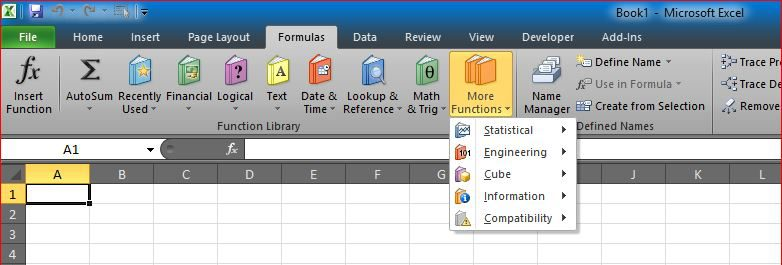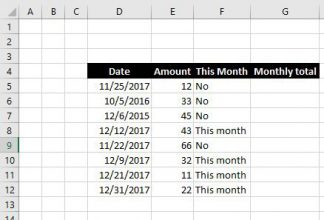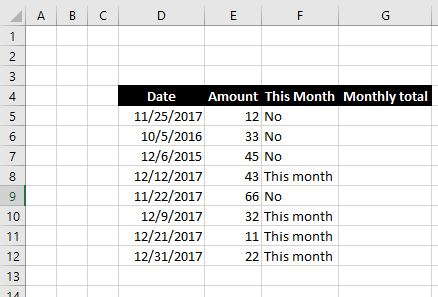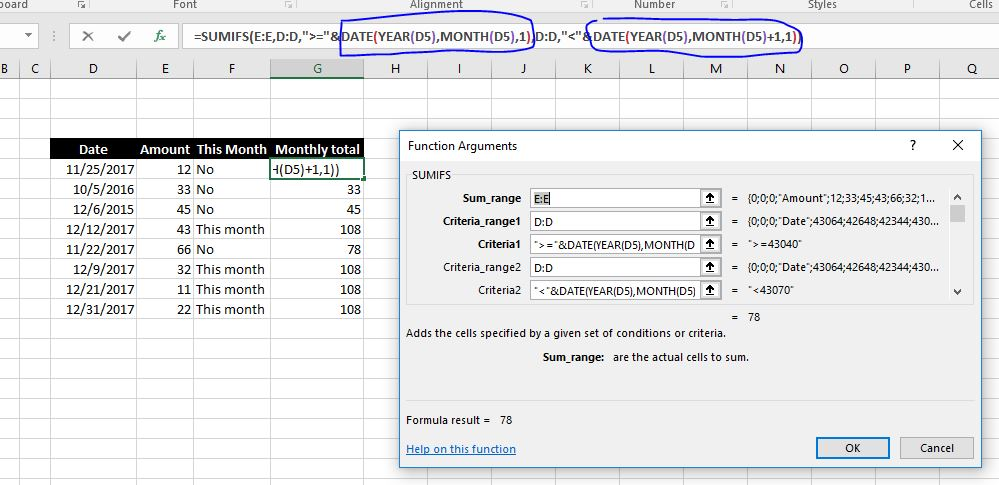# Get monthly total from table with datesAgain, thanks to one of my students, I am getting another post with example 🙂
I did promise her to post it after she sounded interesting in getting this example

Here is an example on how to calculate total amounts of dollars per month (and number of days) from a table with dates

The tricky part is dates, you need to get total by month, while you have dates, and not months, it does not seam to have a direct way

### Why SUMIF can’t help?

SUMIF does not help here, because it only works for one condition, we need two

We need to get total of a certain column if date is between 1st of month, and end of month, which actually are two conditions and not one

### So, how can we do that?

When we have a table like belowAnd we need to get the monthly total of amount, in column in G

SUMIFS is the way to do that, it was added in Excel2010, I would guess based on demand?

Anyways, SUMIFS works like SUMIF, but it can handle multiple conditions, up to 256, with AND.

Means it will get the total of a column, if (and only if) all conditions are met

The flexibility of SUMIFS gives us a lot of features, as we have it below, SUMIFS (along with other functions like DATE, YEAR, etc.) can get us the monthly total of amount.

`=SUMIFS(E:E,D:D,">="&DATE(YEAR(D5),MONTH(D5),1),D:D,"<"&DATE(YEAR(D5),MONTH(D5)+1,1))`

If this complicated, we will break it down.

We are building the conditions inside SUMIFS, by saying…

If the date is more than or equal first of that month, and date is less than the 1st of next month, then get amount

So we created the two dates, 1st of this month, and 1st of next month, using DATE, with YEAR and MONTH as highlighted in screenshotThen we used & to concatenate the criteria in Criteria1 and Criteria2

Then we asked SUMIFS to do the rest

Anytime you do changes, you can see those numbers updated as we expected

### What if I cannot do SUMIFS?

Of course back in the day before SUMIFS we did another trick

Which is adding the month and year in a separate column, then do the Sum using SUMIF having condition on that column

But that should be in another article …